﻿ 基于数字图像相关和有限元法分析45＃钢悬臂梁在微小外力下的应变场 Analysis of the Strain Field of 45# Cantilever Beam under Tiny External Forces Based on Digital Image Correlation Method and Finite Element Analytic Method

International Journal of Mechanics Research
Vol. 07  No. 03 ( 2018 ), Article ID: 26753 , 10 pages
10.12677/IJM.2018.73011

Analysis of the Strain Field of 45# Cantilever Beam under Tiny External Forces Based on Digital Image Correlation Method and Finite Element Analytic Method

Yizhi Li1, Yuanyuan Zhang2, Jie Xia2

1School of Manufacturing Science and Engineering, Southwest University of Science and Technology, Mianyang Sichuan

2School of Science, Southwest University of Science and Technology, Mianyang Sichuan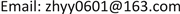Received: Aug. 22nd, 2018; accepted: Sep. 3rd, 2018; published: Sep. 10th, 2018ABSTRACT

This paper uses the digital image correlation method and the finite element analysis to analyze the strain field of 45# steel cantilever beam. Firstly, the strain fields of the cantilever beam are calculated employing MATLAB 2D digital image correlation software Ncorr, and the corresponding analyses are given. Then, simulation results of the strain fields of the tested materials were carried out by using the model building software Pro/E and the Static Mechanical module under the finite element calculation commercial software Ansys 15.0. Comparing the calculation results with the simulation results, it can be seen that the results of Ncorr software and commercial software are in good agreement with each other. All of the results show that the farther the observation point is away from the position of the force, the smaller the stress is.

Keywords:Cantilever Beam, Digital Image Correlation, Finite Element Analysis, Strain Analysis

1西南科技大学制造科学与工程学院，四川 绵阳

2西南科技大学理学院，四川 绵阳Copyright © 2018 by authors and Hans Publishers Inc.1. 引言

2. 数字图像相关基本原理

$\left\{\begin{array}{l}x\text{'}=x+u+\frac{\partial u}{\partial x}\Delta x+\frac{\partial u}{\partial y}\Delta y\\ y\text{'}=y+v+\frac{\partial v}{\partial x}\Delta x+\frac{\partial v}{\partial y}\Delta y\end{array}$ (1)

${C}_{f,g}\left(\stackrel{\to }{P}\right)=\sum _{x=-M}^{M}\sum _{y=-M}^{M}\left[\frac{f\left(x,y\right)}{\sqrt{\sum _{x=-M}^{M}\sum _{y=-M}^{M}{f}^{2}\left(x,y\right)}}-\frac{g\left(x,y\right)}{\sqrt{\sum _{x=-M}^{M}\sum _{y=-M}^{M}{g}^{2}\left(x,y\right)}}\right]$ (2)

3. 试验测量与分析

3.1. 数字图像测量系统和试验材料介绍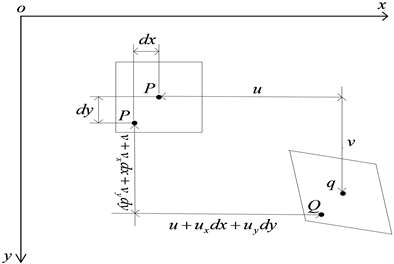Figure 1. Diagram of image subareas before and after deformation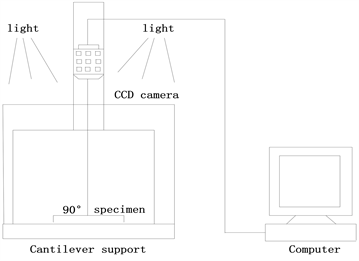Figure 2. Digital image measurement system

3.2. 实验具体过程

1) 人工散斑：在实验开始前需要对样本喷洒人工散斑，具体做法为在试样表面喷上一薄层白色油漆作为底色，然后再喷上黑色油漆，从而得到黑白人工散斑。

2) 固定样本：实验中悬臂梁固定端四点固定在点胶机上，其中第一颗螺母距离梁的边缘为40 mm，第二颗螺母距离边缘为112 mm，第一排螺母与第二排螺母间距72 mm，实验中在矩形梁自由端施加5 N的外力，同时采集施加负荷前后的图像。其装置示意图如图3所示。

3) 实验参数设置：数字图像采集后，利用Ncorr软件对位移场和应变场进行分析。利用Ncorr软件计算应变场时，从参考图像中选择一个子集，并在变形图像中跟踪其相应位置。具体计算中，设置圆形子集半径和子集间距为110 pix和4 pix,，应力半径为100 pix。

3.3. 测量结果与分析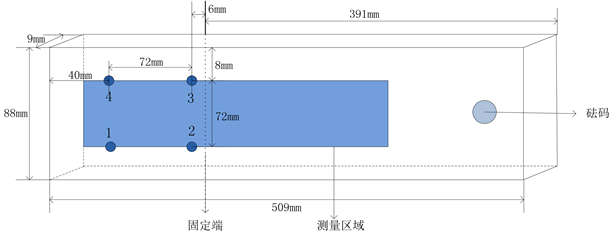Figure 3. Sample image collection diagram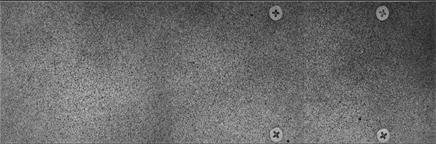Figure 4. Image before loading (reference image)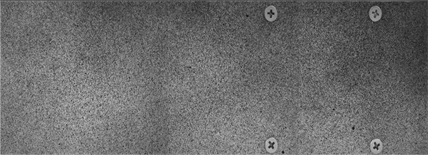Figure 5. Image after loading (target image)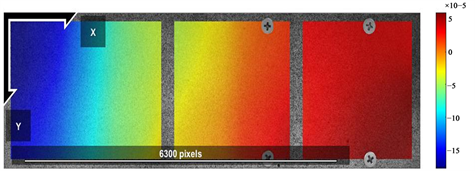Figure 6. Trend of ${E}_{xx}$ in Ncorr software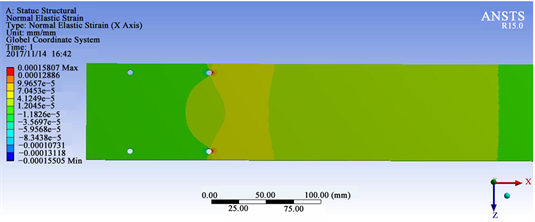Figure 7. Trend of in ansys R15.0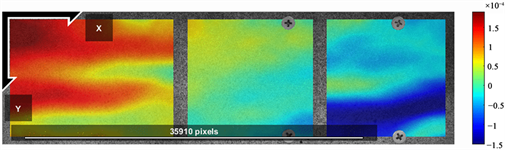Figure 8. Trend of ${E}_{yy}$ in Ncorr software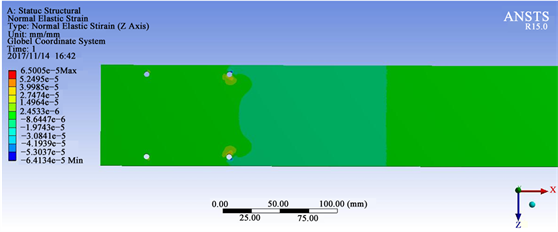Figure 9. Trend of in ansys R15.0

10b和图11b，分别探讨了ROI左边界，离ROI左边界 $\frac{1}{3}$ ，离ROI左边界 $\frac{2}{3}$ 处，ROI右边界条件下45#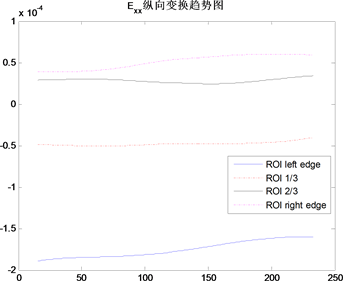(a)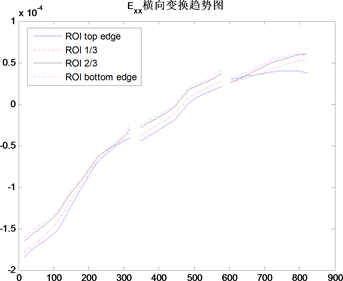(b)

Figure 10. Trend of ${E}_{xx}$ Transverse (a) and ${E}_{xx}$ Lengthwise (b)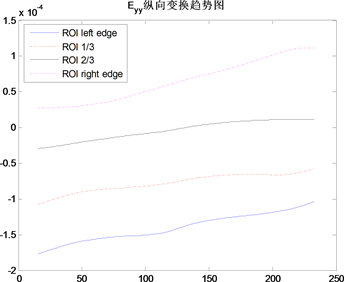(a)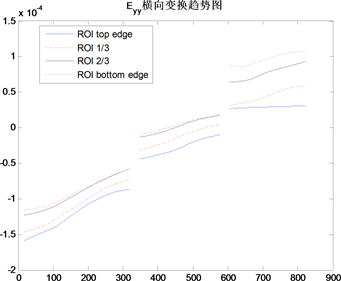(b)

Figure 11. Trend of ${E}_{yy}$ Transverse (a) and ${E}_{yy}$ Lengthwise (b)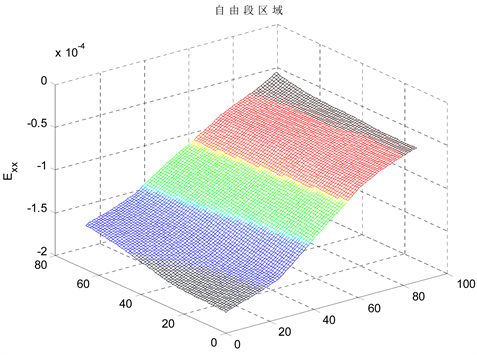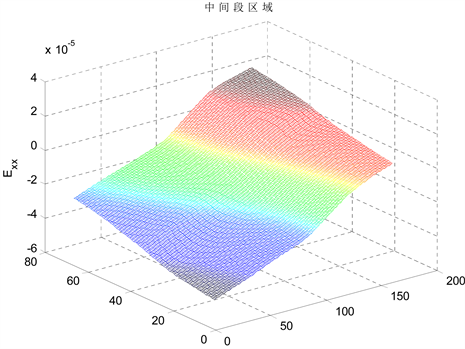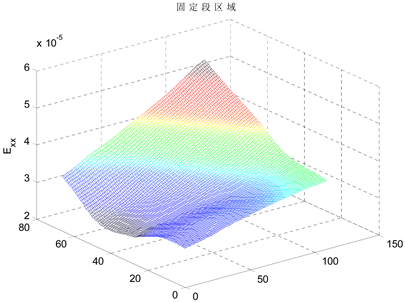Figure 12. ${E}_{xx}$ Strain situation in different regions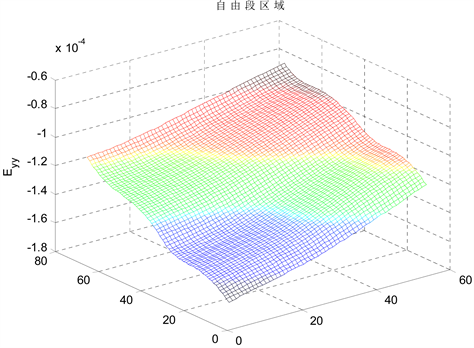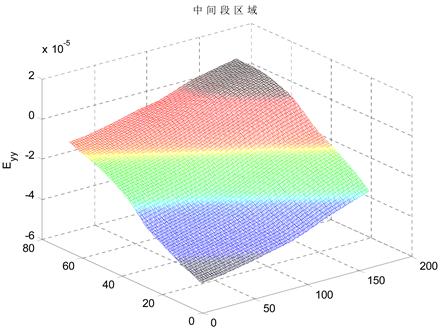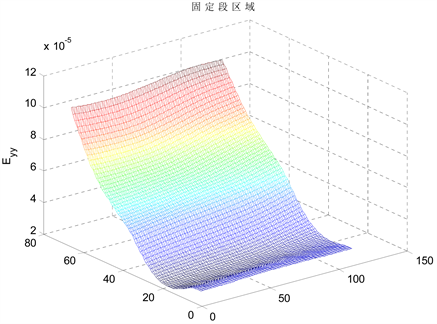Figure 13. ${E}_{yy}$ Strain situation in different regions

4. 结论

Analysis of the Strain Field of 45# Cantilever Beam under Tiny External Forces Based on Digital Image Correlation Method and Finite Element Analytic Method[J]. 力学研究, 2018, 07(03): 95-104. https://doi.org/10.12677/IJM.2018.73011

1. 1. Zhang, D., Zhang, X. and Cheng, G. (1999) Compression Strain Measurement by Digital Speckel Correction. Experimental Mechanics, 39, 62-65. https://doi.org/10.1007/BF02329302

2. 2. Peter, W.H. and Ranson, W.F. (1981) Digital Imaging Techniques in Experimental Stress Analysis. Optical Engineering, 21, 427-431.

3. 3. 张金奎, 李亚智, 张丽, 等. 基于移动最小二乘拟合的数字图像相关应变测量[J]. 科学技术与工程, 2010, 10(3): 686-691.

4. 4. 梁瑜, 梁晋, 王晓光, 等. 采用数字图像相关法的人体测量[J]. 长春理工大学学报(自然科学版), 2017, 40(2): 127-131.

5. 5. Jiang, J.W. and Ni, F.J. (2017) Evaluation of Fatigue Property of Asphalt Mixtures Based on Digital Image Correlation Method. Journal of Southeast University (English Edition), 33, 216-223.

6. 6. Yuan, J., Fan, B., Wan, Y., et al. (2008) The Control Technique and the Finite Element Analysis (FEA) of Active Polishing Lap Based on the ANSYS. World Automation Congress, WAC, Hawaii, HI, 28 September-2 October 2008, 1-4.

7. 7. 杨帆, 宋小军. 悬臂梁的有限元分析[J]. 科技广场, 2008, 25(5): 131-132.

8. 8. 黄鹏刚, 卢林枫, 张顺. 带悬臂梁段拼接的异型节点弱轴连接的抗震性能有限元分析[J]. 建筑技术, 2017, 48(4): 371-374.

9. 9. Saint-Venant de AJCB. (1855) Mémoiresur la torsion des prismes, Mémoiresprésentes pars divers Savants à 1’Académie des Sciences de 1’Institut Impérial de France. Vol. 14, 233-560.